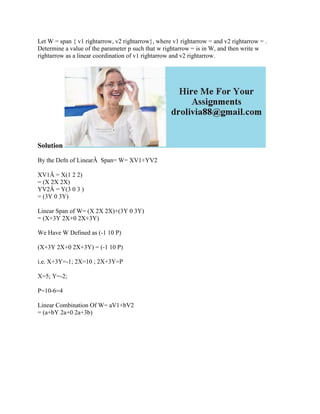Ce diaporama a bien été signalé.
Le téléchargement de votre SlideShare est en cours. ×

# Let W - span { v1 rightarrow- v2 rightarrow}- where v1 rightarrow - a.docx

Publicité
Publicité
Publicité
Publicité
Publicité
Publicité
Publicité
Publicité
Publicité
Publicité
PublicitéChargement dans…3
×

## Consultez-les par la suite

1 sur 2 Publicité

# Let W - span { v1 rightarrow- v2 rightarrow}- where v1 rightarrow - a.docx

Let W = span { v1 rightarrow, v2 rightarrow}, where v1 rightarrow = and v2 rightarrow = . Determine a value of the parameter p such that w rightarrow = is in W, and then write w rightarrow as a linear coordination of v1 rightarrow and v2 rightarrow.
Solution
By the Defn of LinearÂ  Span= W= XV1+YV2
XV1Â = X(1 2 2)
= (X 2X 2X)
YV2Â = Y(3 0 3 )
= (3Y 0 3Y)
Linear Span of W= (X 2X 2X)+(3Y 0 3Y)
= (X+3Y 2X+0 2X+3Y)

We Have W Defined as (-1 10 P)
(X+3Y 2X+0 2X+3Y) = (-1 10 P)

i.e. X+3Y=-1; 2X=10 ; 2X+3Y=P
X=5; Y=-2;
P=10-6=4
Linear Combination Of W= aV1+bV2
= (a+bY 2a+0 2a+3b)
.

Let W = span { v1 rightarrow, v2 rightarrow}, where v1 rightarrow = and v2 rightarrow = . Determine a value of the parameter p such that w rightarrow = is in W, and then write w rightarrow as a linear coordination of v1 rightarrow and v2 rightarrow.
Solution
By the Defn of LinearÂ  Span= W= XV1+YV2
XV1Â = X(1 2 2)
= (X 2X 2X)
YV2Â = Y(3 0 3 )
= (3Y 0 3Y)
Linear Span of W= (X 2X 2X)+(3Y 0 3Y)
= (X+3Y 2X+0 2X+3Y)

We Have W Defined as (-1 10 P)
(X+3Y 2X+0 2X+3Y) = (-1 10 P)

i.e. X+3Y=-1; 2X=10 ; 2X+3Y=P
X=5; Y=-2;
P=10-6=4
Linear Combination Of W= aV1+bV2
= (a+bY 2a+0 2a+3b)
.

Publicité
Publicité

### Let W - span { v1 rightarrow- v2 rightarrow}- where v1 rightarrow - a.docx

1. 1. Let W = span { v1 rightarrow, v2 rightarrow}, where v1 rightarrow = and v2 rightarrow = . Determine a value of the parameter p such that w rightarrow = is in W, and then write w rightarrow as a linear coordination of v1 rightarrow and v2 rightarrow. Solution By the Defn of LinearÂ Span= W= XV1+YV2 XV1Â = X(1 2 2) = (X 2X 2X) YV2Â = Y(3 0 3 ) = (3Y 0 3Y) Linear Span of W= (X 2X 2X)+(3Y 0 3Y) = (X+3Y 2X+0 2X+3Y) We Have W Defined as (-1 10 P) (X+3Y 2X+0 2X+3Y) = (-1 10 P) i.e. X+3Y=-1; 2X=10 ; 2X+3Y=P X=5; Y=-2; P=10-6=4 Linear Combination Of W= aV1+bV2 = (a+bY 2a+0 2a+3b)